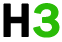C++基础 专题

C++ 异常处理

• throw: 当问题出现时，程序会抛出一个异常。这是通过使用 throw 关键字来完成的。
• catch: 在您想要处理问题的地方，通过异常处理程序捕获异常。catch 关键字用于捕获异常。
• try: try 块中的代码标识将被激活的特定异常。它后面通常跟着一个或多个 catch 块。

```try
{
// 保护代码
}catch( exceptionname e1 )
{
// catch 块
}catch( exceptionname e2 )
{
// catch 块
}catch( exceptionname en )
{
// catch 块
}
```

## 抛出异常

```double division(int a, int b)
{
if( b == 0 )
{
throw "division by zero condition!";
}
return (a/b);
}
```

## 捕获异常

catch 块跟在 try 块后面，用于捕获异常。您可以指定想要捕捉的异常类型，这是由 catch 关键字后的括号内的异常声明决定的。

```try
{
// 保护代码
}catch( exceptionname e )
{
// 处理 exceptionname 异常的代码
}
```

```try
{
// 保护代码
}catch(...)
{
// 能处理任何异常的代码
}
```

``````#include <iostream>
using namespace std;

double division(int a, int b)
{
if( b == 0 )
{
throw "division by zero condition!";
}
return (a/b);
}

int main ()
{
int x = 50;
int y = 0;
double z = 0;

try {
z = division(x, y);
cout << z << endl;
}catch (const char* msg) {
cerr << msg << endl;
}

return 0;
}``````

```division by zero condition!
```

## c++ 标准的异常

c++ 提供了一系列标准的异常，定义在 <exception> 中，我们可以在程序中使用这些标准的异常。它们是以父子类层次结构组织起来的，如下所示：

std::exception 该异常是所有标准 c++ 异常的父类。
std::logic_error 理论上可以通过读取代码来检测到的异常。
std::domain_error 当使用了一个无效的数学域时，会抛出该异常。
std::invalid_argument 当使用了无效的参数时，会抛出该异常。
std::length_error 当创建了太长的 std::string 时，会抛出该异常。
std::out_of_range 该异常可以通过方法抛出，例如 std::vector 和 std::bitset<>::operator[]()。
std::runtime_error 理论上不可以通过读取代码来检测到的异常。
std::overflow_error 当发生数学上溢时，会抛出该异常。
std::range_error 当尝试存储超出范围的值时，会抛出该异常。
std::underflow_error 当发生数学下溢时，会抛出该异常。

## 定义新的异常

``````#include <iostream>
#include <exception>
using namespace std;

struct myexception : public exception
{
const char * what () const throw ()
{
return "c++ exception";
}
};

int main()
{
try
{
throw myexception();
}
catch(myexception& e)
{
std::cout << "myexception caught" << std::endl;
std::cout << e.what() << std::endl;
}
catch(std::exception& e)
{
//其他的错误
}
}``````

```myexception caught
c++ exception
```